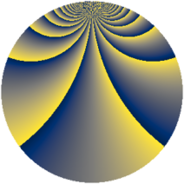# Properties

 Label 2304.3.bfLevel $2304$ Weight $3$ Character orbit 2304.bf Rep. character $\chi_{2304}(271,\cdot)$ Character field $\Q(\zeta_{16})$ Dimension $632$ Sturm bound $1152$

# Related objects

## Defining parameters

 Level: $$N$$ $$=$$ $$2304 = 2^{8} \cdot 3^{2}$$ Weight: $$k$$ $$=$$ $$3$$ Character orbit: $$[\chi]$$ $$=$$ 2304.bf (of order $$16$$ and degree $$8$$) Character conductor: $$\operatorname{cond}(\chi)$$ $$=$$ $$64$$ Character field: $$\Q(\zeta_{16})$$ Sturm bound: $$1152$$

## Dimensions

The following table gives the dimensions of various subspaces of $$M_{3}(2304, [\chi])$$.

Total New Old
Modular forms 6272 648 5624
Cusp forms 6016 632 5384
Eisenstein series 256 16 240

## Trace form

 $$632q + 8q^{5} + 8q^{7} + O(q^{10})$$ $$632q + 8q^{5} + 8q^{7} - 8q^{11} - 8q^{13} + 8q^{17} + 8q^{19} - 8q^{23} - 8q^{25} + 8q^{29} + 16q^{31} - 8q^{35} - 8q^{37} + 8q^{41} + 8q^{43} - 8q^{47} - 8q^{49} + 8q^{53} - 504q^{55} + 248q^{59} - 8q^{61} + 16q^{65} - 56q^{67} - 520q^{71} - 8q^{73} + 8q^{77} + 520q^{79} - 8q^{83} - 8q^{85} + 8q^{89} + 8q^{91} - 16q^{95} + O(q^{100})$$

## Decomposition of $$S_{3}^{\mathrm{new}}(2304, [\chi])$$ into newform subspaces

The newforms in this space have not yet been added to the LMFDB.

## Decomposition of $$S_{3}^{\mathrm{old}}(2304, [\chi])$$ into lower level spaces

$$S_{3}^{\mathrm{old}}(2304, [\chi]) \cong$$ $$S_{3}^{\mathrm{new}}(64, [\chi])$$$$^{\oplus 9}$$$$\oplus$$$$S_{3}^{\mathrm{new}}(192, [\chi])$$$$^{\oplus 6}$$$$\oplus$$$$S_{3}^{\mathrm{new}}(256, [\chi])$$$$^{\oplus 3}$$$$\oplus$$$$S_{3}^{\mathrm{new}}(576, [\chi])$$$$^{\oplus 3}$$$$\oplus$$$$S_{3}^{\mathrm{new}}(768, [\chi])$$$$^{\oplus 2}$$